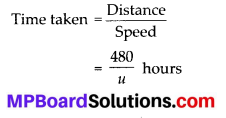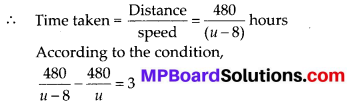# MP Board Class 10th Maths Solutions Chapter 4 Quadratic Equations Ex 4.1

In this article, we will share MP Board Class 10th Maths Book Solutions Chapter 4 Quadratic Equations Ex 4.1 Pdf, These solutions are solved subject experts from the latest edition books.

## MP Board Class 10th Maths Solutions Chapter 4 Quadratic Equations Ex 4.1

Question 1.
Check whether the following are quadratic equations:
(i) (x + 1 )2 = 2(x – 3)
(ii) x2 – 2x = (-2)(3 – x)
(iii) (x – 2)(x + 1) = (x – 1 )(x + 3)
(iv) (x – 3)(2x + 1) = x(x + 5)
(v) (2x – 1 )(x – 3) = (x + 5)(x – 1)
(vi) x2 + 3x + 1 = (x – 2)2
(vii) (x + 2)3 = 2x(x2 – 1)
(viii) x3 – 4x2 – x + 1 = (x – 2)3
Solution:
(i) We have, (x + 1)2 = 2(x – 3)
⇒ x2 + 2x + 1 = 2x – 6
⇒ x2 + 2x + 1 – 2x + 6 = 0
⇒ x2 + 7 = 0
Since, x2 + 7 is a quadratic polynomial.
∴ (x + 1)2 = 2(x – 3) is a quadratic equation.

(ii) We have, x2 – 2x = (-2) (3 – x)
⇒ x2 – 2x = – 6 + 2x
⇒ x2 – 2x – 2x + 6 = 0
⇒ x2 – 4x + 6 = 0
Since, x2 – 4x + 6 is a quadratic polynomial
∴ x2 – 2x = (-2)(3 – x) is a quadratic equation.

(iii) We have, (x – 2)(x + 1) = (x – 1) (x + 3)
⇒ x2 – x2 = x2 + 2x3
⇒ x2 – x – 2 – x2 – 2x + 3 = 0
⇒ – 3x +1 = 0
Since, – 3x + 1 is a linear polynomial.
∴ (x – 2)(x + 1) = (x – 1)(x + 3) is not a quadratic equation.(iv) We have, (x – 3) (2x + 1) = x(x + 5)
⇒ 2x2 + x – 6x – 3 = x2 + 5x
⇒ 2x2 – 5x – 3 – x2 – 5x = 0
⇒ x2 – 10x – 3 = 0
Since, x2 – 10x – 3 is a quadratic polynomial.
∴ (x – 3)(2x + 1) = x(x + 5) is a quadratic equation.

(v) We have, (2x – 1) (x – 3) = (x + 5) (x – 1)
⇒ 2x2 – 6x – x + 3 = x2 – x + 5x – 5
⇒ 2x2 – 7x + 3 = x2 + 4x – 5
⇒ 2x2 – 7x + 3 – x2 – 4x + 5 = 0
⇒ x2 – 11x + 8 = 0
Since, x2 – 11x + 8 is a quadratic polynomial
∴ (2x – 1)(x – 3) = (x + 5)(x -1) is a quadratic equation.

(vi) We have, x2 + 3x + 1 = (x – 2)2
⇒ x2 + 3x + 1 = x2 – 4x + 4
⇒ x2 + 3x + 1 – x2 + 4x – 4 = 0
⇒ 7x – 3 = 0
Since, 7x – 3 is a linear polynomial.
x2 + 3x + 1 = (x – 2)2 is not a quadratic equation.(vii) We have, (x + 2)3 = 2x(x2 – 1)
⇒ x3 + 3x2(2) + 3x(2)2 + (2)3 = 2x3 – 2x
⇒ x3 + 6x2 + 12x + 8 = 2x3 – 2x
⇒ x3 + 6x2 + 12x + 8 – 2x3 + 2x = 0
⇒ -x3 + 6x2 + 14x + 8 = 0
Since, -x3 + 6x2 + 14x + 8 is a polynomial of degree 3.
∴ (x + 2)3= 2x(x2 – 1) is not a quadratic equation.

(viii) We have, x3 – 4x2 – x + 1 = (x – 2)3
⇒ x3 – 4x2 – x + 1 = x3 + 3x2(-2) + 3x(-2)2 + (-2)3
⇒ x3 – 4x2 – x + 1 = x3 – 6x2 + 12x – 8
⇒ x3 – 4x2 – x + 1 – x3 + 6x2 – 12x + 8 = 0 ⇒ 2x2 – 13x + 9 = 0
Since, 2x2 – 13x + 9 is a quadratic polynomial.
∴ x3 – 4x2 – x + 1 = (x – 2)3 is a quadratic equation.Question 2.
Represent the following situations in the form of quadratic equations:
(i) The area of a rectangular plot is 528 m2. The length of the plot (in metres) is one more than twice its breadth. We need to find the length and breadth of the plot.
(ii) The product of two consecutive positive integers is 306. We need to find the integers.
(iii) Rohan’s mother is 26 years older than him. The product of their ages (in years) 3 years from now will be 360. We would like to find Rohan’s present age.
(iv) A train travels a distance of 480 km at a uniform speed. If the speed had been 8 km/hr less, then it would have taken 3 hours more to cover the same distance. We need to find the speed of the train.
Solution:
(i) Let the breadth = x metres
∵ Length = 2(Breadth) + 1
∴ Length = (2x + 1)metres
Since, Length × Breadth = Area
∴ (2x + 1) × x = 528 ⇒ 2x2 + x = 528
⇒ 2x2 + x – 528 = 0
Thus, the required quadratic equation is 2x2 + x – 528 = 0

(ii) Let the two consecutive positive integers be x and (x + 1).
∵ Product of two consecutive positive integers = 306
∴ x(x + 1) = 306 ⇒ x2 + x = 306
⇒ x2 + x – 306 = 0
Thus, the required quadratic equation is x2 + x – 306 = 0

(iii) Let the present age of Rohan be x years
∴ Mother’s age = (x + 26) years
After 3 years,
Rohan’s age = (x + 3) years
Mother’s age = [(x + 26) + 3] years
= (x + 29) years
According to the condition,
(x + 3) × (x + 29) = 360
⇒ x2 + 29x + 3x + 87 = 360
⇒ x2 + 29x + 3x + 87 – 360 = 0
⇒ x2 + 32x – 273 = 0
Thus, the required quadratic equation is x2 + 32x – 273 = 0

(iv) Let the speed of the train = u km/hr Distance covered = 480 km DistanceIn second case,
Speed = (u – 8) km/hour⇒ 480u – 480(u – 8) = 3u(u – 8)
⇒ 480u – 480u + 3840 = 3u2 – 24u
⇒ 3840 – 3u2 + 24u = 0
⇒ u2 – 8u – 1280 = 0
Thus, the required quadratic equation is u2 – 8u – 1280 = 0